# 2D Line Plot in MATLAB

• Last Updated : 29 May, 2021

‘2D’ stands for 2-dimensional and a 2D line is a line that is moved in 2-dimensions. A line in 2D means that we could move in forward and backward direction but also in any direction like left, right, up, down.

In MATLAB we have a function named plot() which allows us to plot a line in 2 directions.

Syntax:

plot(X,Y)

where X and Y represent the x and the y axis of the plane. The X and Y both are can be vectors or matrices but there are some conditions to plot the graph and these conditions are mentioned below:

Condition 1: If X and Y both are vectors then they must be of equal length.

Condition 2: If X and Y both are Matrices then they must be of equal size.

Condition 3: If one of X or Y is a vector and the other is a matrix, then the matrix must have dimensions such that one of its dimensions equals the vector length.

Condition 4: If one is a scalar and the other is either a scalar or a vector, then discrete points must be plotted.

Now let’s move to some examples.

Example 1: Draw a simple line:

## MATLAB

 `% coordinates of the x-axis``x=[10,20,30,40,50]` `% coordinates of the y-axis``y=[100,200,300,400,500]` `% plot function is used to plot the``% line according to the coordinates``plot(x,y)``  ` `% to put grid on the graph``grid on`

Output :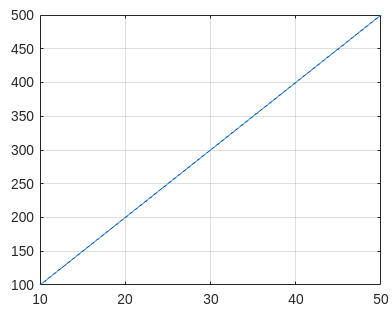Example 2: Draw a line with only 1 axis coordinates as input:

Note: If you give only 1 axis then the plot() function takes it as coordinates of the y-axis and by default gives values to the x-axis starting from 1, 2, 3, up to y coordinates.

## MATLAB

 `% coordinates of axis``y=[100,200,300,400,500]` `% plot function is used to plot the``% line according to the coordinates``plot(x,y)` `% to put grid on the graph``grid on`

Output :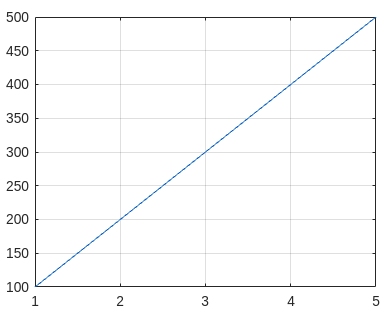Example 3: Draw more than 1 line on the same graph with axis names:

## MATLAB

 `% coordinates of x-axis``x=[10,20,30,40,50]` `% coordinates of y-axis of line 1``% represented by blue color``y1=[100,500,200,100,0]` `% coordinates of y-axis of line 2``% represented by red color``y2=[400,100,0,200,300]` `% coordinates of y-axis of line 3``% represented by yellow color``y3=[200,300,400,100,500]` `% plot function to plot the lines on graph``plot(x,y1,x,y2,x,y3)` `% to add grid on graph``grid on` `% name of x axis``xlabel(``'x'``)``  ` `% name of y axis``ylabel(``'y'``)`

Output :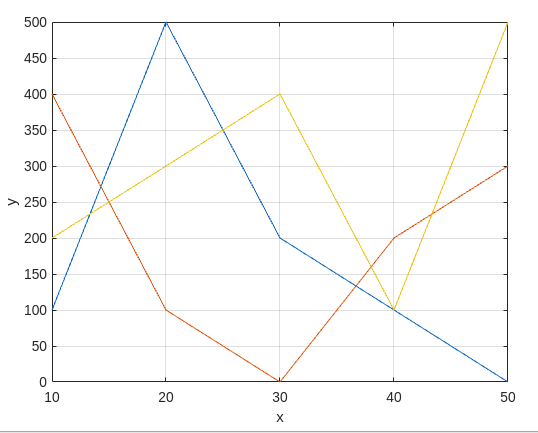Example 4: Now the value of the y-axis is given as matrix instead of vector:

## MATLAB

 `% coordinates of x-axis``x=[1,2,3,4,5]` `% coordinates of y-axis in form of matrix``% magic(n) matrix is a n*n matrix``% in which value scattered from 1 to n^2``% with equal row and columns sum``y=magic(5)` `% plot function``plot(x,y)` `% to add grid``grid on` `% add name on axis``xlabel(``'x'``)``ylabel(``'y'``)`

Output: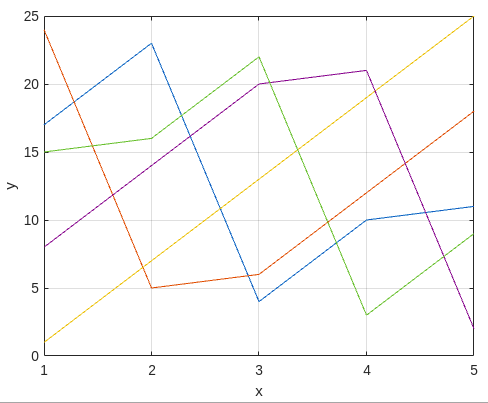Example 5: Now we plot the graph by using 2 y-axis one is on the left side another is on the right side.

## MATLAB

 `% coordinates of x-axis``x=[1,2,3,4,5]` `% coordinates of y-axis``y=[50,40,30,20,10]` `% assigning left side to the above``% coordinates``yyaxis left` `% plot graph of left y-axis``plot(x,y)``  ` `% coordinates of y-axis``y=[10,20,30,40,50]` `% assigning right side to the above``% coordinates``yyaxis right` `% plot graph of right y-axis``plot(x,y)` `% put grid on graph``grid on` `% name of x-axis``xlabel(``'x'``)``  ` `% name of left side y coordinates``yyaxis left``ylabel(``'Left Side'``)``  ` `% name of right side y coordinates``yyaxis right``ylabel(``'Right Side'``)`

Output: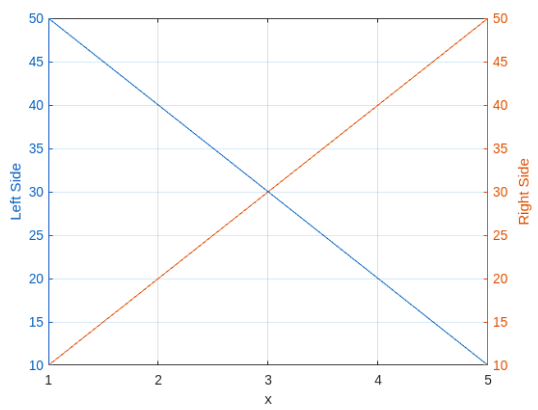My Personal Notes arrow_drop_up